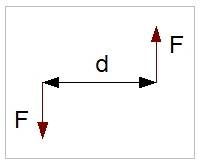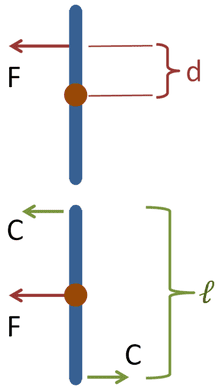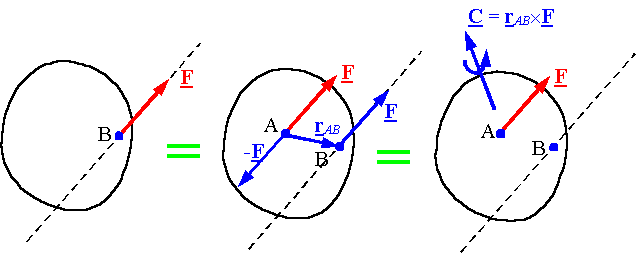# What is moment actually?

Hii guys,i'm having problem in understanding momen.
is it a effect of particular physical quantity at some distance like effect of force on some other point where it is not acting?
N if it is,then why it shows effect of force 0 on where force is acting bcoz force have some effect on where it actsacts,certainly.i wanna know its physical significant.
Much confused...

Last edited:

The moment of a force F about the origin is defined as
M = r x F
where
M = the resulting moment
r = radius vector from the origin to the point where the force is applied
F = the force.
If you know the definition of a cross product, this should give you what you need.

jack action
Gold Member
A moment is the tendency of a force to rotate an object about an axis.

Every force creates a moment about any axis you can imagine.

One way of looking at it is to imagine that if you isolate a force F and you want to evaluate the moment it produces about an arbitrary axis in space at a distance d then it must have an opposite and equal force acting at that axis such that the system is in equilibrium. The moment is the resultant of those two forces acting one against each other some distance apart.

But if the distance between the force and the axis is null, then the two forces just go one against the other and no moment is created, i.e. the force cannot induce a rotation about that axis.OldEngr63
Gold Member
Jack, you figure looks like you intended to talk about a couple, but that is beyond the scope of the present discussion. This figure confuses the issue as to which force F is causing a moment about what point.

jack action
Gold Member
Jack, you figure looks like you intended to talk about a couple, but that is beyond the scope of the present discussion. This figure confuses the issue as to which force F is causing a moment about what point.

That was kind of my point to help see why there is an «effect of force on some other point where it is not acting».

What I'm saying is that you could imagine that in a system of forces where you want to know the resultant moment about a certain axis, each force can be seen as some sort of couple with its opposite force acting at the axis you chose. This way the resultant force is the same and you can see how a force can «effect at some other point it is not acting».

Another way of looking at it that you might appreciate more is to say that every force not acting at the center of mass has the same effect as the same force applied directly to the center of mass and an equivalent couple where Cℓ = Fd:That was my understanding of the OP's problem but I might be wrong.

That's nice of you, jack sir,but i believe it's not that moment is always about forces.we take moment of other physical quantities too.like moment of inertia show the resistance offered by mass when it's motion tends to change at some other point in space where it is not located actually.
So i think it always show effect of a physical quantity at some other point.
So i want to know a definition of moment,which describes moment of every physical quantity and not just of force.
Thank you all for your support and understanding.

SteamKing
Staff Emeritus
Homework Helper
So i want to know a definition of moment,which describes moment of every physical quantity and not just of force.

Just replace the 'force' quantity in the moment formula with whatever other physical quantity (area, volume, weight, etc.) for which you want to determine the moment. The units of the resulting moment must be adjusted to suit.

@SteamKing ,So i can say like its a effect of a quantity at any point

Last edited:
SteamKing
Staff Emeritus
Homework Helper
@streamking ,So i can say like its a effect of a quantity at any point
It's Steam, not Stream.

I don't know what you mean by 'effect of a quantity at any point'.

The moment has a defined meaning and magnitude about an axis, not a point. If you change the axis, you change the magnitude of the resulting moment.

The best way I understand it is to think of a torque. Torque is a force applied over a distance that causes twisting. When you think of moment think of torque, they essentially are the same thing.

I'm sorry for that @SteamKing.
consider a situation of opening a gate.at one end we apply q force and at other end a reaction force acts on it too,equal in magnitude and opposite in direction. Its center of gravity will be at its center.this point moves,with gate(when gate rotate) but resultant force on it is zero as it is center of gravity,but it moves anyway?? how is that??first explain it please.

jack action
Gold Member
consider a situation of opening a gate.at one end we apply q force and at other end a reaction force acts on it too,equal in magnitude and opposite in direction. Its center of gravity will be at its center.this point moves,with gate(when gate rotate) but resultant force on it is zero as it is center of gravity,but it moves anyway?? how is that??first explain it please.

This is exactly what I explained previously:

Another way of looking at it that you might appreciate more is to say that every force not acting at the center of mass has the same effect as the same force applied directly to the center of mass and an equivalent couple where Cℓ = Fd:The blue line is your gate from the top view. The «offset» force you put on the gate (with respect to the mass center) can be replaced by a force of the same magnitude acting at the mass center with the addition of a couple Cℓ.

SteamKing
Staff Emeritus
Homework Helper
This is exactly what I explained previously:

The blue line is your gate from the top view. The «offset» force you put on the gate (with respect to the mass center) can be replaced by a force of the same magnitude acting at the mass center with the addition of a couple Cℓ.

I think the OP is getting confused by your second diagram, jackaction. When you illustrate the couple applied to the bar, the diagram implies that that there is a force acting at the center of the bar. This is not true. Since the forces applied at the ends of the bar are equal in magnitude but are acting in the opposite direction to one another, the net force acting on the gate is zero. The only thing left is the couple, or moment, which acts around the axis running thru the screen into (or out of) the center of the bar.

In the upper diagram, one may replace the force F acting a distance d from the center of the bar with the same force acting at the center of the bar plus a moment whose magnitude is F * d, also acting about an axis thru the center of the bar, perpendicular to the screen.

jack action
Gold Member
When you illustrate the couple applied to the bar, the diagram implies that that there is a force acting at the center of the bar. This is not true.

Yes, it is.

If Wikipedia is not good enough for reference, University of Nebraska-Lincoln has something similar:Here's a nice video about it:

OldEngr63
Gold Member
So i want to know a definition of moment,which describes moment of every physical quantity and not just of force.

You are asking too much; this is not possible. It is just a fact of the way that language works that the word "moment" has more than one meaning.

In the context of physics, most folks think of moment as referring to a torque, as in all the discussion by Jack and SteamKing. But you are correct that it is also used in the context of "moment of inertia" where it simply means something different altogether.

We also talk about the moment of an area, meaning
int(r*da) taken over an area.
This may be confusing, but that's the breaks. It is the way the language has developed over the centuries, and if you want to play the game, you have to speak the language as it is spoken.